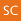### Metode Nonlinear Least Square (NLS) untuk Estimasi Parameter Model Wavelet Radial Basis Neural Network (WRBNN)

*Rukun Santoso-  Departemen Statistika, Fakultas Sains dan Matematika, Universitas Diponegoro, Indonesia
Sudarno Sudarno  -  Departemen Statistika, Fakultas Sains dan Matematika, Universitas Diponegoro, IndonesiaCopyright (c) 2017 MEDIA STATISTIKA

Citation Format:
Article Info
Section: Articles
Language: EN
Statistics: 643 791
Abstract

The use of wavelet radial basis model for forecasting nonlinear time series is introduced in this paper. The model is generated by artificial neural network approximation under restriction that the activation function on the hidden layers is radial basis. The current model is developed from the multiresolution autoregressives (MAR) model, with addition of radial basis function in the hidden layers. The power of model is compared to the other nonlinear model existed before, such as MAR model and Generalized Autoregressives Conditional Heteroscedastic (GARCH) model. The simulation data which be generated from GARCH process is applied to support the aim of research. The sufficiency of model is measured by sum squared of error (SSE). The computation results show that the proposed model has a power as good as GARCH model to carry on the heteroscedastic process.

Keywords:

Wavelet, Radial Basis, Heteroscedastic Model, Neural Network Model.

Article Metrics: